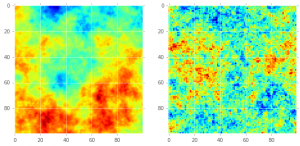# Stationary GMRF simulation with Discrete Fourier Transformation

### Algorithm to simulate GMRF with block-circulant Matrix.

Algorithm 2.10

Taken from: Rue, H., & Held, L. (2005). Gaussian Markov random fields: theory and applications. CRC press.

### let’s build the circulant matrix for the tourus

Oke, for the moment I´ll follow the example in GMRF book. i.e. a Torus (stationary of 128×128)

def createToroidalCircularBase(d=0.1,N=128):
"""
Creates a circular base similar to the one described in GMRF Rue and Held, 2005.
"""
c00 = np.hstack(((4 + d),-1,np.zeros(N - 3),-1))
c01 = np.hstack((-1,np.zeros(N - 1)))
c0 = np.zeros((N - 2 ,N))
c1 = np.vstack((c00,c01))
c = np.vstack((c1,c0))
c[N -1, 0] = -1
return c



%%time
## Create circular base
d = 0.00001
N = 100
c = createToroidalCircularBase(d=d,N=N)
## Simulate random noise (Normal distributed)
from scipy.fftpack import ifft2, fft2
zr = scipy.stats.norm.rvs(size=(c.size,2),loc=0,scale=1,random_state=1234)
zr.dtype=np.complex_
#plt.hist(zr.real)
#Lm = scipy.sqrt(C.shape*C.shape) * fft2(C)

Lm = fft2(c)
v = 1.0/ len(c) * fft2((Lm ** -0.5)* zr.reshape(Lm.shape))
x = v.real
plt.imshow(x,interpolation='None')
Both figures represent a simulation on a 100×100 cells. The comparison is as follows: on the right there is full gaussian field with mean zero and some Matern-based covariance structure. On the right a Markov Random Field simulation using the method described above.## [Bonus] Create a simple precision matrix

An easy way to create a precision matrix is with the function: *diags* inside the module: scipy.sparse. The code will look like this:

from scipy.sparse import diags
N = 10
diagonals = [np.zeros(N), np.ones(N-1), np.ones(N-1)]
# order 0 := diagonal , 1 first on top of diagonal, -1 first bottom of diagonal
offset = [0,1,-1]
## maps diagonals with position (offset)
M = diags(diagonals,offset).toarray()
## "seal the edges"
M = 1
M[N-1][N-1] =1
print(M)

Result:

[[ 1.  1.  0.  0.  0.  0.  0.  0.  0.  0.]
[ 1.  0.  1.  0.  0.  0.  0.  0.  0.  0.]
[ 0.  1.  0.  1.  0.  0.  0.  0.  0.  0.]
[ 0.  0.  1.  0.  1.  0.  0.  0.  0.  0.]
[ 0.  0.  0.  1.  0.  1.  0.  0.  0.  0.]
[ 0.  0.  0.  0.  1.  0.  1.  0.  0.  0.]
[ 0.  0.  0.  0.  0.  1.  0.  1.  0.  0.]
[ 0.  0.  0.  0.  0.  0.  1.  0.  1.  0.]
[ 0.  0.  0.  0.  0.  0.  0.  1.  0.  1.]
[ 0.  0.  0.  0.  0.  0.  0.  0.  1.  1.]]

## Update

There is a new tutorial for simulating a CAR model using a given adjacency matrix and spatial lattice. Go here to read it.